# Entropy Formula

## Entropy Formula

A large element of chance is inherited in the natural processes. For example, the spacing between trees is a random natural process. Likewise, falling of tree leaves on the ground with the random arrangement is also a random process. Entropy is the measure of disorders or randomness of the particular system. Since it depends on the initial and final state of the system, the absolute value of entropy cannot be determined. You need to consider the difference between initial and final state to determine the change in entropy.

The change in Entropy Formula is expressed as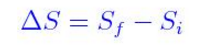According to thermodynamic definition, entropy is based on change in entropy (ds) during physical or chemical changes and expressed as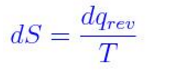For change to be measurable between initial and final state, the integrated expression is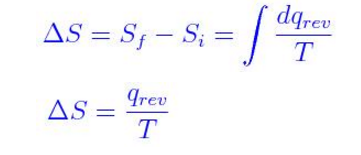The units for entropy is calories per degree or Cal deg-1. Molar Entropy is  written in joules per kelvin per mole (JK-1mol-1)

Example 1

Determine the entropy change per mole for ice which melts at 0oC. Note that the change in enthalpy is equal to the heat supplied to the system at constant pressure. ΔH (fusion) = +6.01Kj/mol

Solution

The equation can be expressed as

H2O(s) —> H2O(l)

ΔH (fusion) = +6.01Kj/mol

You can use the equation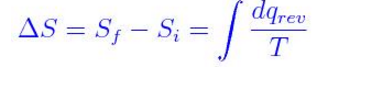ΔS = 6010 J Mol-1/273K

= +22.0 JK-1mol-1

Example 2

Determine ΔS for synthesis of ammonia at 25oc.

N2 + 3H2 —> 2NH3

ΔH = -92.6kJ/mol

Solution

We can use the formula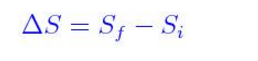ΔS = 2(NH3) – [S(N2) + 3S(H2)]

ΔS = (2)(192.5 JK-1mol-1) – [191.6JK-1mol-1 + (3)(130.6 JK-1mol-1)]

ΔS = -198.4 JK-1mol-1